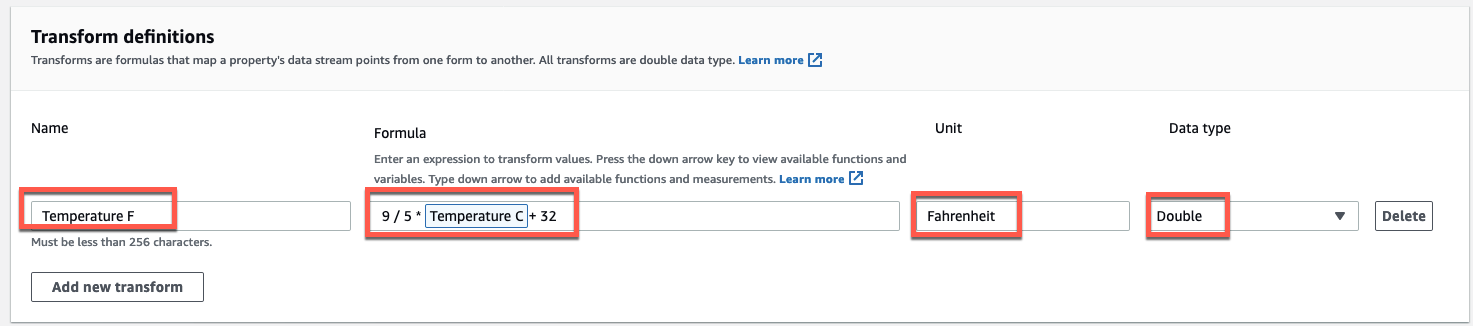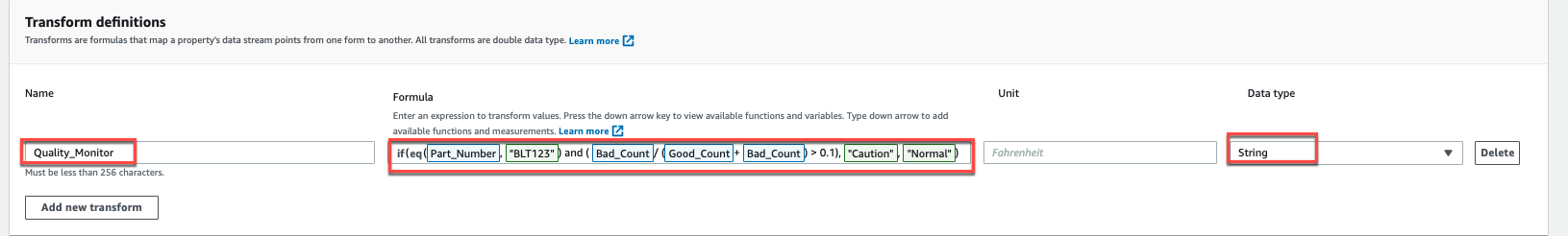Transforming data (transforms) - Amazon IoT SiteWise
Services or capabilities described in Amazon Web Services documentation might vary by Region. To see the differences applicable to the China Regions, see Getting Started with Amazon Web Services in China (PDF).

# Transforming data (transforms)

Transforms are mathematical expressions that map asset properties' data points from one form to another. A transform expression consists of asset property variables, literals, operators, and functions. The transformed data points hold a one-to-one relationship with the input data points. Amazon IoT SiteWise calculates a new transformed data point each time any of the input properties receives a new data point.

For example, if your asset has a temperature measurement stream named `Temperature_C` with units in Celsius, you can convert each data point to Fahrenheit with the formula `Temperature_F = 9/5 * Temperature_C + 32`. Each time Amazon IoT SiteWise receives a data point in the `Temperature_C` measurement stream, the corresponding `Temperature_F` value is calculated within a few seconds and available as the `Temperature_F` property.

If your transform contains more than one variable, the data point that arrives earlier initiates the computation immediately. Consider an example where a parts manufacturer uses a transform to monitor product quality. Using a different standard based on the part type, the manufacturer uses the following measurements to represent the process:

• `Part_Number` ‐ A string that identifies the part type.

• `Good_Count` ‐ An integer that increases by one if the part meets the standard.

• `Bad_Count` ‐ An integer that increases by one if the part doesn't meet the standard.

The manufacturer also creates a transform, `Quality_Monitor`, that equals ``` if(eq(Part_Number, "BLT123") and (Bad_Count / (Good_Count + Bad_Count) > 0.1), "Caution", "Normal")```.

This transform monitors the percentage of bad parts produced for a specific part type. If the part number is BLT123 and the percentage of bad parts exceeds 10 percent (0.1), the transform returns `"Caution"`. Otherwise, the transform returns `"Normal"`.

Note
• If `Part_Number` receives a new data point before other measurements, the `Quality_Monitor` transform uses the new `Part_Number` value and the latest `Good_Count` and `Bad_Count` values. To avoid errors, reset `Good_Count` and `Bad_Count` before the next manufacturing run.

• Use metrics if you want to evaluate expressions only after all variables receive new data points.

## Defining transforms (console)

When you define a transform for an asset model in the Amazon IoT SiteWise console, you specify following parameters:

• Name – The property's name.

• Formula – The transform expression. Transform expressions can't use aggregation functions or temporal functions. Start typing or press the down arrow key to open the auto complete feature. For more information, see Using formula expressions.

Important

Transforms can input properties that are integer, double, Boolean, or string type. Booleans convert to `0` (false) and `1` (true).

Transforms must input one or more properties that aren't attributes and any number of attribute properties. Amazon IoT SiteWise calculates a new transformed data point each time the input property that isn't an attribute receives a new data point. New attribute values don't trigger transform updates. The same request rate for asset property data API operations applies for transform computation results.

Formula expressions can only output double or string values. Nested expressions can output other data types, such as strings, but the formula as a whole must evaluate to a number or string. You can use the jp function to convert a string to a number. The Boolean value must be 1 (true) or 0 (false). For more information, see Undefined, infinite, and overflow values.

• Unit – (Optional) The scientific unit for the property, such as mm or Celsius.

• Data type – The data type of the transform, which can be Double or String.

###### Example transform definition

The following example demonstrates a transform property that converts an asset's temperature measurement data from Celsius to Fahrenheit.###### Example transform definition that contains three variables

The following example demonstrates a transform property that returns a warning message (`"Caution"`) if more than 10 percent of the BLT123 parts don't meet the standard. Otherwise, it returns an information message (`"Normal"`).## Defining transforms (CLI)

When you define a transform for an asset model with the Amazon IoT SiteWise API, you specify the following parameters:

• `name` – The property's name.

• `dataType` – The data type of the transform, which must be `DOUBLE` or `STRING`.

• `expression` – The transform expression. Transform expressions can't use aggregation functions or temporal functions. For more information, see Using formula expressions.

• `variables` – The list of variables that defines the other properties of your asset to use in the expression. Each variable structure contains a simple name to use in the expression and a `value` structure that identifies which property to link to that variable. The `value` structure contains the following information:

• `propertyId` – The ID of the property from which to input values. You can use the property's name instead of its ID.

Important

Transforms can input properties that are integer, double, Boolean, or string type. Booleans convert to `0` (false) and `1` (true).

Transforms must input one or more properties that aren't attributes and any number of attribute properties. Amazon IoT SiteWise calculates a new transformed data point each time the input property that isn't an attribute receives a new data point. New attribute values don't trigger transform updates. The same request rate for asset property data API operations applies for transform computation results.

Formula expressions can only output double or string values. Nested expressions can output other data types, such as strings, but the formula as a whole must evaluate to a number or string. You can use the jp function to convert a string to a number. The Boolean value must be 1 (true) or 0 (false). For more information, see Undefined, infinite, and overflow values.

• `unit` – (Optional) The scientific unit for the property, such as mm or Celsius.

###### Example transform definition

The following example demonstrates a transform property that converts an asset's temperature measurement data from Celsius to Fahrenheit. This object is an example of an AssetModelProperty that contains a Transform. You can specify this object as a part of the CreateAssetModel request payload to create a transform property. For more information, see Creating an asset model (CLI).

``````{
...
"assetModelProperties": [
...
{
"name": "Temperature F",
"dataType": "DOUBLE",
"type": {
"transform": {
"expression": "9/5 * temp_c + 32",
"variables": [
{
"name": "temp_c",
"value": {
"propertyId": "Temperature C"
}
}
]
}
},
"unit": "Fahrenheit"
}
],
...
}``````
###### Example transform definition that contains three variables

The following example demonstrates a transform property that returns a warning message (`"Caution"`) if more than 10 percent of the BLT123 parts don't meet the standard. Otherwise, it returns an information message (`"Normal"`).

``````{
...
"assetModelProperties": [
...
{
"name": "Quality_Monitor",
"dataType": "STRING",
"type": {
"transform": {
"expression": "if(eq(Part_Number,"BLT123") and (Bad_Count / (Good_Count + Bad_Count) > 0.1), "Caution", "Normal")",
"variables": [
{
"name": "Part_Number",
"value": {
"propertyId": "Part Number"
}
},
{
"name": "Good_Count",
"value": {
"propertyId": "Good Count"
}
},
{